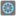• # Why is it printing twice as well as failing checks

## Question related to missionEven the Last

def checkio(array: list) -> int: """ sums even-indexes elements and multiply at the last """ total = 0 for num in range(0,len(array),2): total += num print(total) total = array[::-1] *total return total

# These "asserts" using only for self-checking and not necessary for auto-testing

if name == 'main': print('Example:') print(checkio([0, 1, 2, 3, 4, 5]))

```assert checkio([0, 1, 2, 3, 4, 5]) == 30, "(0+2+4)*5=30"
assert checkio([1, 3, 5]) == 30, "(1+5)*5=30"
assert checkio() == 36, "(6)*6=36"
assert checkio([]) == 0, "An empty array = 0"
print("Coding complete? Click 'Check' to review your tests and earn cool rewards!")
```7